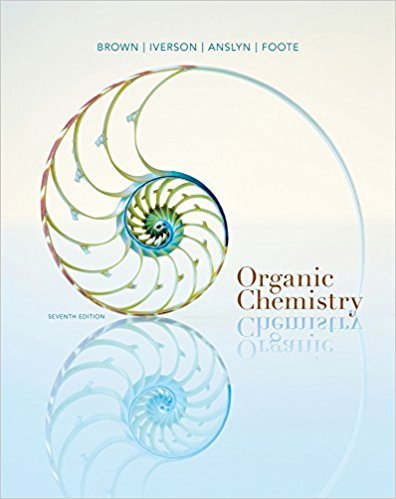×
Get Full Access to Organic Chemistry - 7 Edition - Chapter 18 - Problem 18.61
Get Full Access to Organic Chemistry - 7 Edition - Chapter 18 - Problem 18.61

×

# Suppose that you start with a diisocyanate and a diol. Show how their reaction canISBN: 9781133952848 483

## Solution for problem 18.61 Chapter 18

Organic Chemistry | 7th Edition

• Textbook Solutions
• 2901 Step-by-step solutions solved by professors and subject experts
• Get 24/7 help from StudySoup virtual teaching assistantsOrganic Chemistry | 7th Edition

4 5 1 262 Reviews
24
1
Problem 18.61

Suppose that you start with a diisocyanate and a diol. Show how their reaction can leadto a polymer called a polyurethane (Section 29.5D).

Step-by-Step Solution:
Step 1 of 3

Chemistry 1030 April 26, 2016 Chapter 11 – Gases https://docs.google.com/presentation/d/16UDwxEWD4o8C5cItI6elkonEEoDB3 n_lK9E3B9x-XEY/edit#slide=id.p16 11.1- Properties of Gases  A sample of a gas assumes both the shape and the volume of a container  Gases are compressible  Densities are much smaller than liquids and solids and are variable depending on the temperature and pressure  Gases are always homogenous mixtures with other gases 11.3- Gas Pressure  Pressure- force applied per unit o Pressure = Force/Area o 1 Pa = 1 N/m squared o common standard pressure units-  1 atm = 760 mmHg = 760 torr = 101.3 kPa 11.4 Gas Laws  Boyle’s Law- constant temperature o Pressure decreases, volume increases o Inverse relationship o Ex- balloon in a vacuum will expand o V = 1/P o P1 x V1 = P2 x V2  Charles and Gay-Lussac’s Law- states that the volume of a gas maintained at constant pressure is directly proportional to the absolute temperature of the gas o Example- heat a balloon and it expands. Cool a balloon and it shrinks.  Avagadro’s Law – states that the volume of a sample of gas is directly proportional to the number of moles in the sample at a constant temperature and pressure  Combined gas law- used to solve problems where any or all of the variables changes o look for variables you have and don’t have 11.5 – Ideal Gas Equation  gas laws c

Step 2 of 3

Step 3 of 3

##### ISBN: 9781133952848

This textbook survival guide was created for the textbook: Organic Chemistry, edition: 7. The full step-by-step solution to problem: 18.61 from chapter: 18 was answered by , our top Chemistry solution expert on 03/16/18, 05:02PM. The answer to “Suppose that you start with a diisocyanate and a diol. Show how their reaction can leadto a polymer called a polyurethane (Section 29.5D).” is broken down into a number of easy to follow steps, and 23 words. This full solution covers the following key subjects: . This expansive textbook survival guide covers 24 chapters, and 1131 solutions. Since the solution to 18.61 from 18 chapter was answered, more than 241 students have viewed the full step-by-step answer. Organic Chemistry was written by and is associated to the ISBN: 9781133952848.

Unlock Textbook Solution